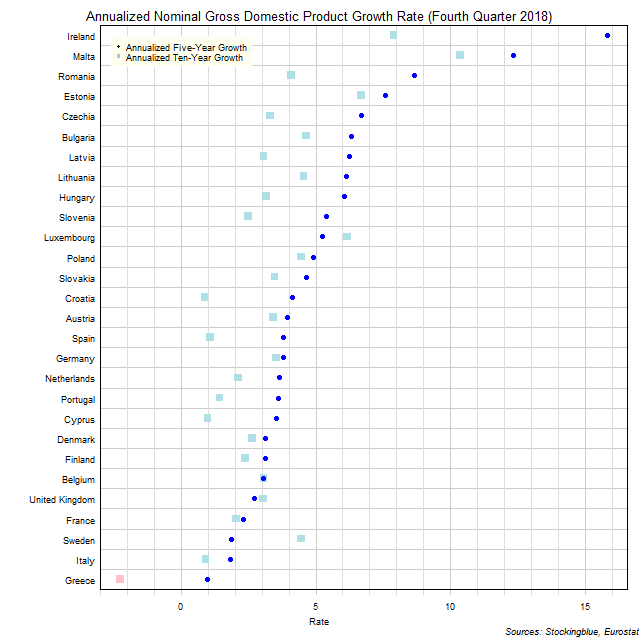# Long-Term EU GDP Growth Rate by State, Fourth Quarter 2018

Jul 31, 2019The chart above shows the annualized nominal gross domestic product (GDP) growth rate in each EU state over the past five years as of the fourth quarter of 2018 and the growth rate over the past ten years.  Greece is the only state to experience negative growth over either period.

## Findings

• The difference between the state with the largest annualized five-year growth rate, Ireland, and the state with the smallest, Greece, is 14.86 percentage points.
• The difference between the state with the largest annualized ten-year growth rate, Malta, and the state with the smallest, Greece, is 12.63 percentage points.
• The median annualized five-year growth rate in the 28 EU states is 4.03% and the mean 5.05%.
• The median annualized ten-year growth rate in the 28 EU states is 3.12% and the mean 3.36%.
• Twenty-four states' economies grew faster over the past five years than they did over the past ten years.  The remaining four states saw their economies grow slower over the past five years than they did over the past ten years.

## Caveats

• Data is from the fourth quarters of 2008, 2013, and 2018.
• The data is seasonally adjusted in current euros.
• The data is annualized by multiplying the quarterly figure by four.
• All figures are rounded to the nearest hundredth.

## Details

Greece had the smallest increase over the past five years with an annualized growth rate of 0.98%.  Ireland had the largest growth with an annualized gain of 15.84%.

Over the past ten years, Greece had the largest decline with an annualized growth rate of -2.27% while Malta had the largest growth with an annualized growth rate of 10.36%.

Sweden saw the largest decrease in its growth rate between its annualized ten-year growth and its annualized five-year growth slowing its rate by 2.60 percentage points.  Ireland had the largest increase in its growth rate between its annualized ten-year growth and its annualized five-year growth ramping up its rate by 7.94 percentage points.

Croatia went from having the 27th largest growth rate over the past ten years to the 14th largest growth rate over the past five years.  Conversely, Sweden went from having the 7th highest growth rate over the past ten years to the 26th highest growth rate over the past five years.  Only three states had annualized growth rates in the top five both over the past ten years and over the past five years: Ireland (2nd highest annualized growth over ten years, highest growth over five years), Malta (highest ten-year, 2nd highest five-year), and Estonia (3rd highest ten-year, 4th highest five-year).  Two states had growth rates in the bottom five both over both periods: Italy (26th ten-year, 27th five-year) and Greece (28th ten-year, 28th five year).

## Sources

Filed under: Charts and Graphs